Скачать презентацию When is The Financing Decision Irrelevant MF 807

• Количество слайдов: 34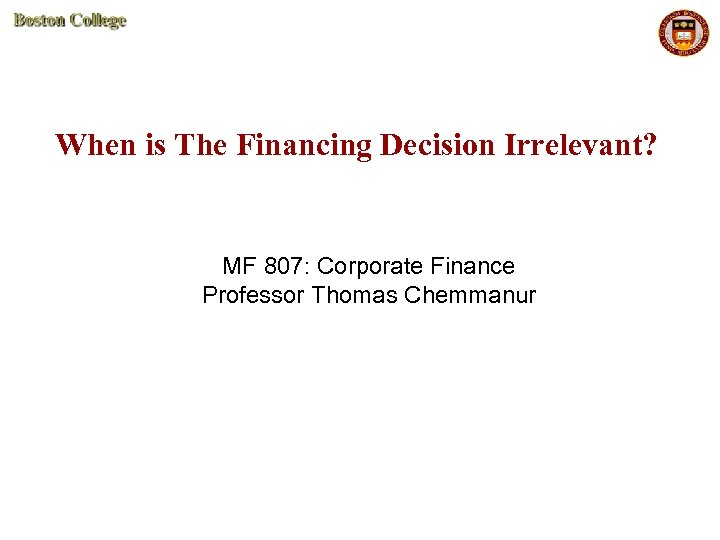When is The Financing Decision Irrelevant? MF 807: Corporate Finance Professor Thomas Chemmanur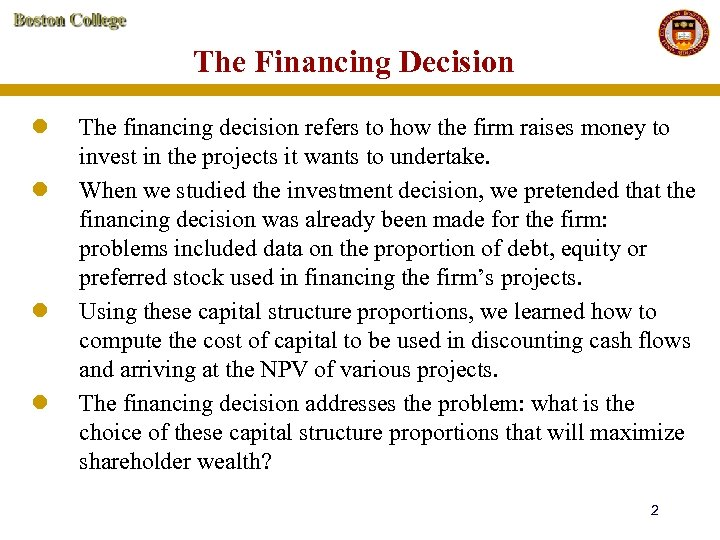The Financing Decision l l The financing decision refers to how the firm raises money to invest in the projects it wants to undertake. When we studied the investment decision, we pretended that the financing decision was already been made for the firm: problems included data on the proportion of debt, equity or preferred stock used in financing the firm’s projects. Using these capital structure proportions, we learned how to compute the cost of capital to be used in discounting cash flows and arriving at the NPV of various projects. The financing decision addresses the problem: what is the choice of these capital structure proportions that will maximize shareholder wealth? 2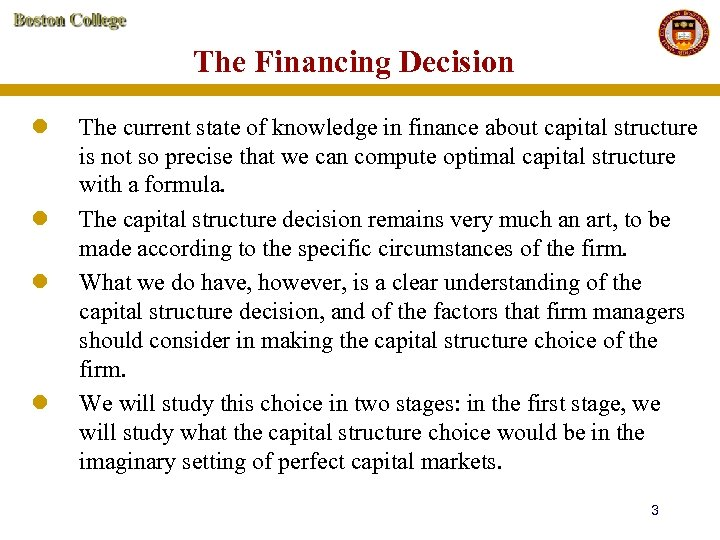The Financing Decision l l The current state of knowledge in finance about capital structure is not so precise that we can compute optimal capital structure with a formula. The capital structure decision remains very much an art, to be made according to the specific circumstances of the firm. What we do have, however, is a clear understanding of the capital structure decision, and of the factors that firm managers should consider in making the capital structure choice of the firm. We will study this choice in two stages: in the first stage, we will study what the capital structure choice would be in the imaginary setting of perfect capital markets. 3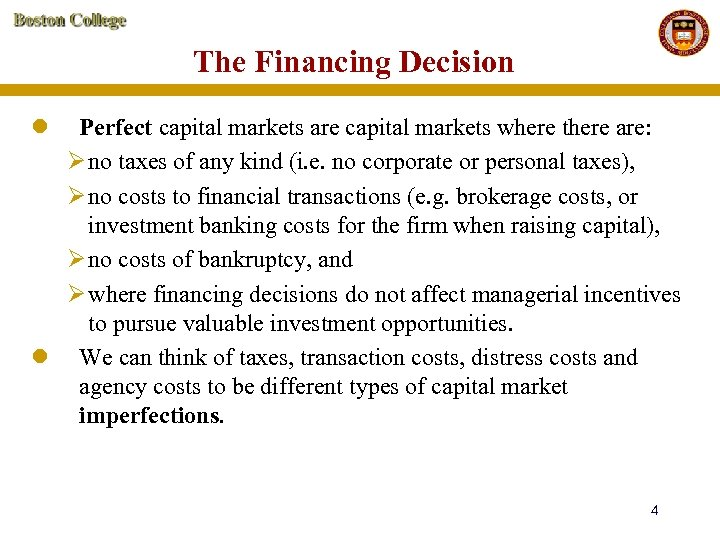The Financing Decision l Perfect capital markets are capital markets where there are: Ø no taxes of any kind (i. e. no corporate or personal taxes), Ø no costs to financial transactions (e. g. brokerage costs, or investment banking costs for the firm when raising capital), Ø no costs of bankruptcy, and Ø where financing decisions do not affect managerial incentives to pursue valuable investment opportunities. l We can think of taxes, transaction costs, distress costs and agency costs to be different types of capital market imperfections. 4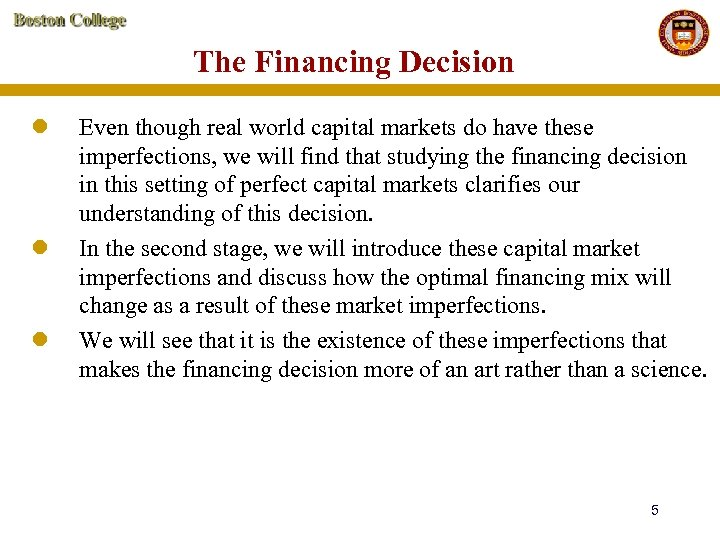The Financing Decision l l l Even though real world capital markets do have these imperfections, we will find that studying the financing decision in this setting of perfect capital markets clarifies our understanding of this decision. In the second stage, we will introduce these capital market imperfections and discuss how the optimal financing mix will change as a result of these market imperfections. We will see that it is the existence of these imperfections that makes the financing decision more of an art rather than a science. 5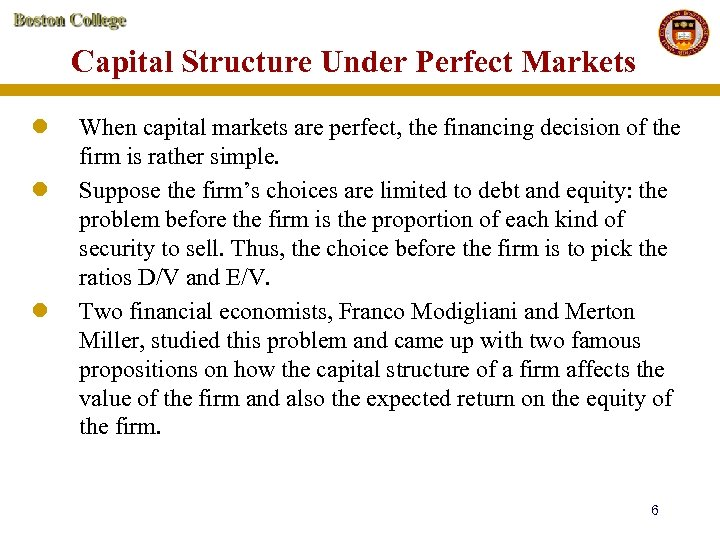Capital Structure Under Perfect Markets l l l When capital markets are perfect, the financing decision of the firm is rather simple. Suppose the firm’s choices are limited to debt and equity: the problem before the firm is the proportion of each kind of security to sell. Thus, the choice before the firm is to pick the ratios D/V and E/V. Two financial economists, Franco Modigliani and Merton Miller, studied this problem and came up with two famous propositions on how the capital structure of a firm affects the value of the firm and also the expected return on the equity of the firm. 6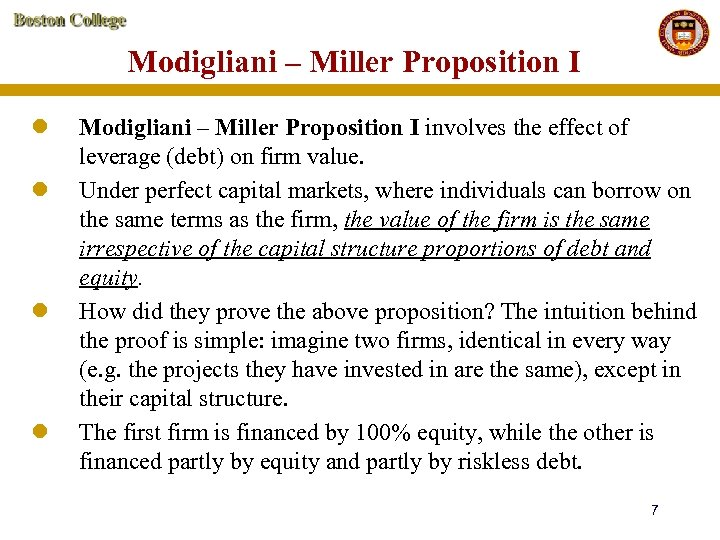Modigliani – Miller Proposition I l l Modigliani – Miller Proposition I involves the effect of leverage (debt) on firm value. Under perfect capital markets, where individuals can borrow on the same terms as the firm, the value of the firm is the same irrespective of the capital structure proportions of debt and equity. How did they prove the above proposition? The intuition behind the proof is simple: imagine two firms, identical in every way (e. g. the projects they have invested in are the same), except in their capital structure. The first firm is financed by 100% equity, while the other is financed partly by equity and partly by riskless debt. 7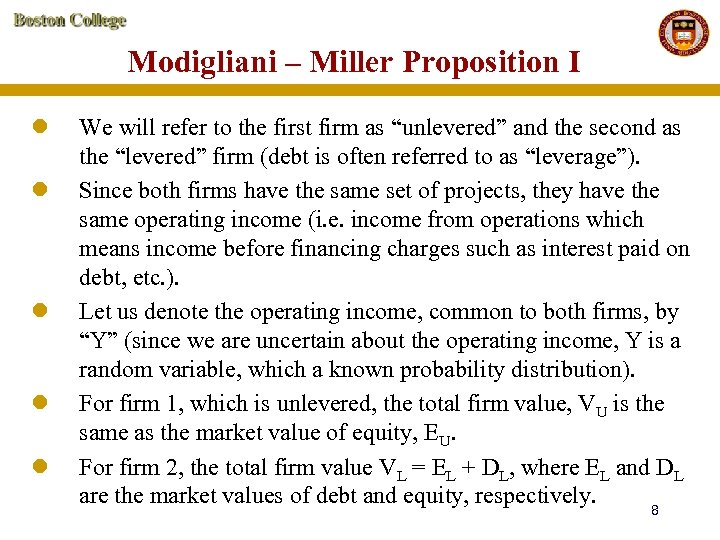Modigliani – Miller Proposition I l l l We will refer to the first firm as “unlevered” and the second as the “levered” firm (debt is often referred to as “leverage”). Since both firms have the same set of projects, they have the same operating income (i. e. income from operations which means income before financing charges such as interest paid on debt, etc. ). Let us denote the operating income, common to both firms, by “Y” (since we are uncertain about the operating income, Y is a random variable, which a known probability distribution). For firm 1, which is unlevered, the total firm value, VU is the same as the market value of equity, EU. For firm 2, the total firm value VL = EL + DL, where EL and DL are the market values of debt and equity, respectively. 8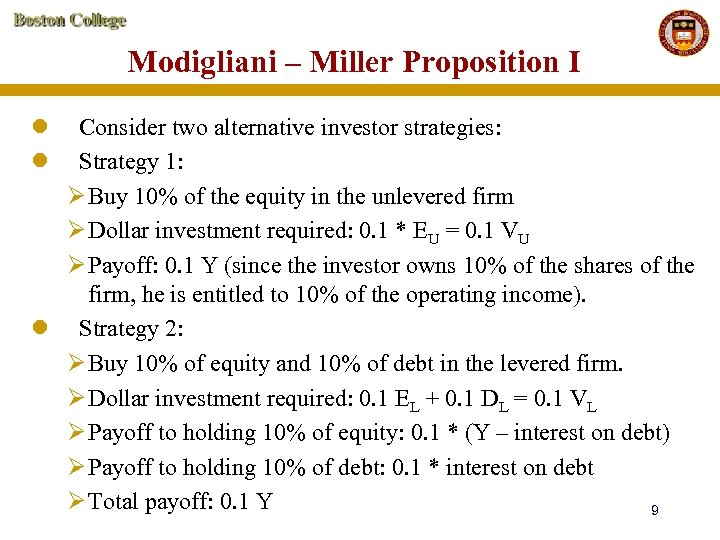Modigliani – Miller Proposition I l l Consider two alternative investor strategies: Strategy 1: Ø Buy 10% of the equity in the unlevered firm Ø Dollar investment required: 0. 1 * EU = 0. 1 VU Ø Payoff: 0. 1 Y (since the investor owns 10% of the shares of the firm, he is entitled to 10% of the operating income). l Strategy 2: Ø Buy 10% of equity and 10% of debt in the levered firm. Ø Dollar investment required: 0. 1 EL + 0. 1 DL = 0. 1 VL Ø Payoff to holding 10% of equity: 0. 1 * (Y – interest on debt) Ø Payoff to holding 10% of debt: 0. 1 * interest on debt Ø Total payoff: 0. 1 Y 9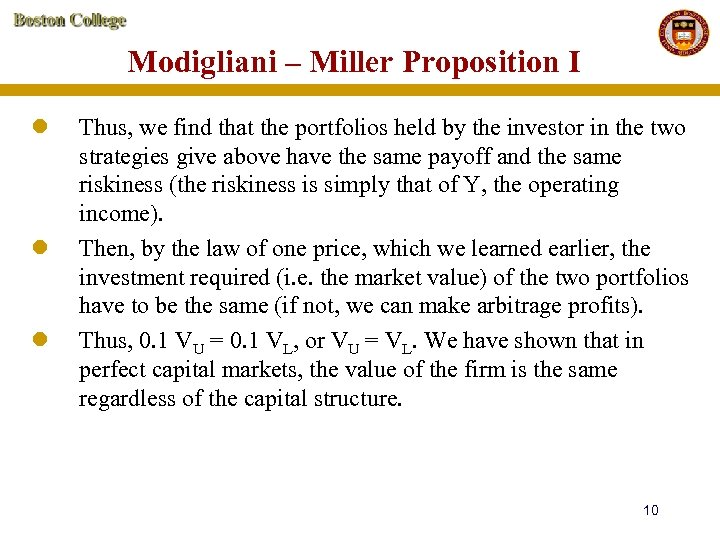Modigliani – Miller Proposition I l l l Thus, we find that the portfolios held by the investor in the two strategies give above have the same payoff and the same riskiness (the riskiness is simply that of Y, the operating income). Then, by the law of one price, which we learned earlier, the investment required (i. e. the market value) of the two portfolios have to be the same (if not, we can make arbitrage profits). Thus, 0. 1 VU = 0. 1 VL, or VU = VL. We have shown that in perfect capital markets, the value of the firm is the same regardless of the capital structure. 10Modigliani – Miller Proposition I l l l We can illustrate Proposition I, using a simple numerical example. Consider two firms, as in the case above, which are identical in every respect except in their capital structure. Let the distribution of their operating income per year be as follows: Unlevered firm: Y 1, 500, 000 0. 5 1, 100, 000 l Probability 0. 5 Therefore, expected operating income per year = 1, 500, 000 (0. 5) + 1, 100, 000 (0. 5) = 1, 300, 000 11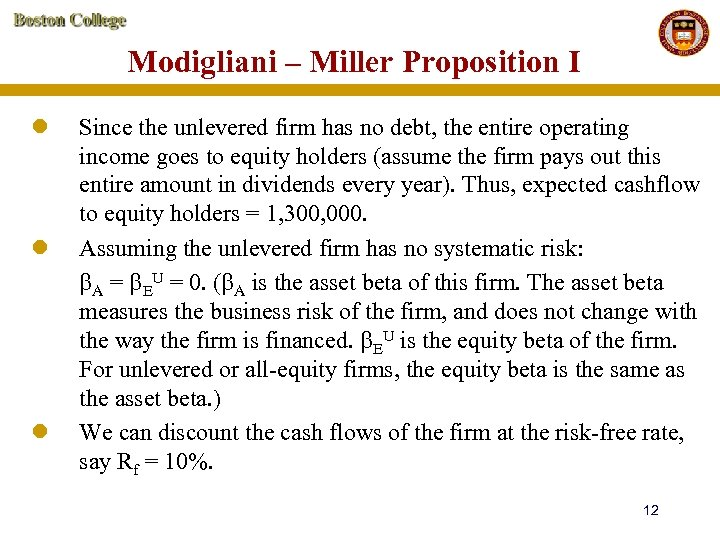Modigliani – Miller Proposition I l l l Since the unlevered firm has no debt, the entire operating income goes to equity holders (assume the firm pays out this entire amount in dividends every year). Thus, expected cashflow to equity holders = 1, 300, 000. Assuming the unlevered firm has no systematic risk: A = EU = 0. ( A is the asset beta of this firm. The asset beta measures the business risk of the firm, and does not change with the way the firm is financed. EU is the equity beta of the firm. For unlevered or all-equity firms, the equity beta is the same as the asset beta. ) We can discount the cash flows of the firm at the risk-free rate, say Rf = 10%. 12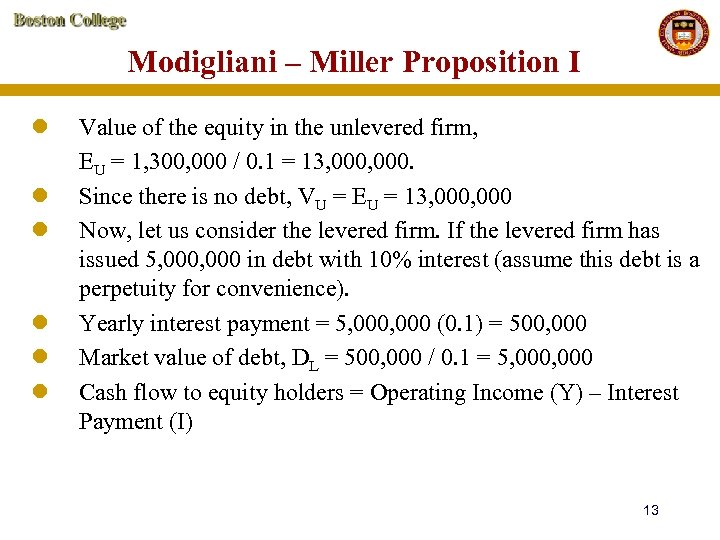Modigliani – Miller Proposition I l l l Value of the equity in the unlevered firm, EU = 1, 300, 000 / 0. 1 = 13, 000. Since there is no debt, VU = EU = 13, 000 Now, let us consider the levered firm. If the levered firm has issued 5, 000 in debt with 10% interest (assume this debt is a perpetuity for convenience). Yearly interest payment = 5, 000 (0. 1) = 500, 000 Market value of debt, DL = 500, 000 / 0. 1 = 5, 000 Cash flow to equity holders = Operating Income (Y) – Interest Payment (I) 13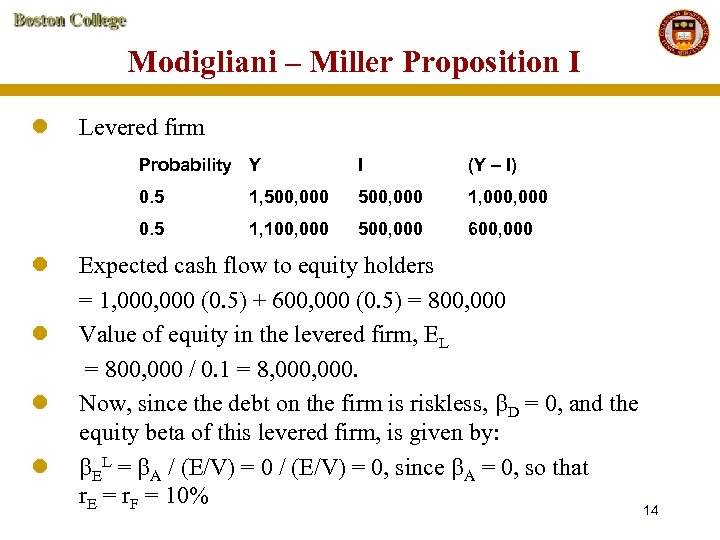Modigliani – Miller Proposition I l Levered firm Probability Y l l 1, 500, 000 1, 000 0. 5 l (Y – I) 0. 5 l I 1, 100, 000 500, 000 600, 000 Expected cash flow to equity holders = 1, 000 (0. 5) + 600, 000 (0. 5) = 800, 000 Value of equity in the levered firm, EL = 800, 000 / 0. 1 = 8, 000. Now, since the debt on the firm is riskless, D = 0, and the equity beta of this levered firm, is given by: EL = A / (E/V) = 0, since A = 0, so that r. E = r. F = 10% 14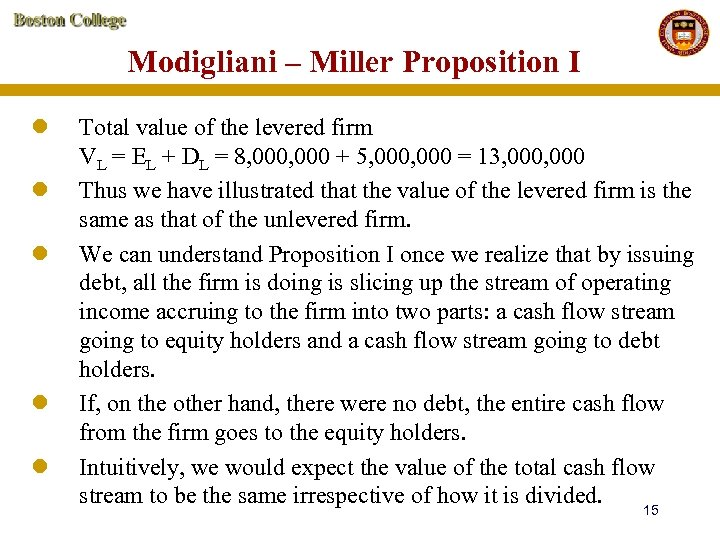Modigliani – Miller Proposition I l l l Total value of the levered firm VL = EL + DL = 8, 000 + 5, 000 = 13, 000 Thus we have illustrated that the value of the levered firm is the same as that of the unlevered firm. We can understand Proposition I once we realize that by issuing debt, all the firm is doing is slicing up the stream of operating income accruing to the firm into two parts: a cash flow stream going to equity holders and a cash flow stream going to debt holders. If, on the other hand, there were no debt, the entire cash flow from the firm goes to the equity holders. Intuitively, we would expect the value of the total cash flow stream to be the same irrespective of how it is divided. 15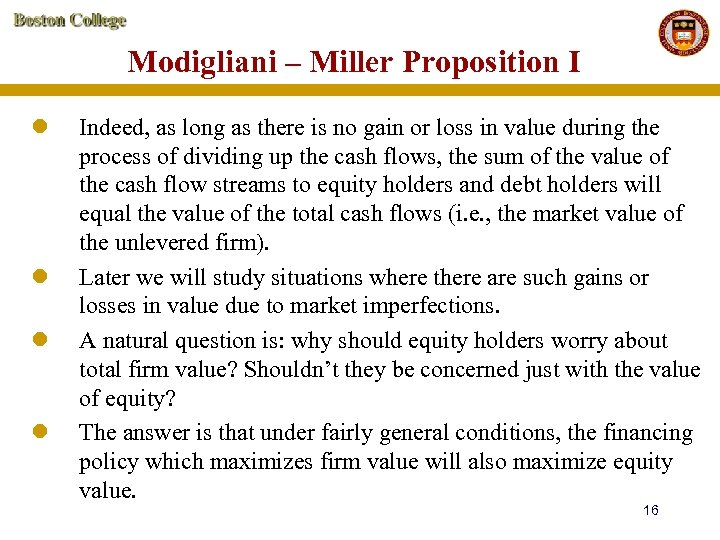Modigliani – Miller Proposition I l l Indeed, as long as there is no gain or loss in value during the process of dividing up the cash flows, the sum of the value of the cash flow streams to equity holders and debt holders will equal the value of the total cash flows (i. e. , the market value of the unlevered firm). Later we will study situations where there are such gains or losses in value due to market imperfections. A natural question is: why should equity holders worry about total firm value? Shouldn’t they be concerned just with the value of equity? The answer is that under fairly general conditions, the financing policy which maximizes firm value will also maximize equity value. 16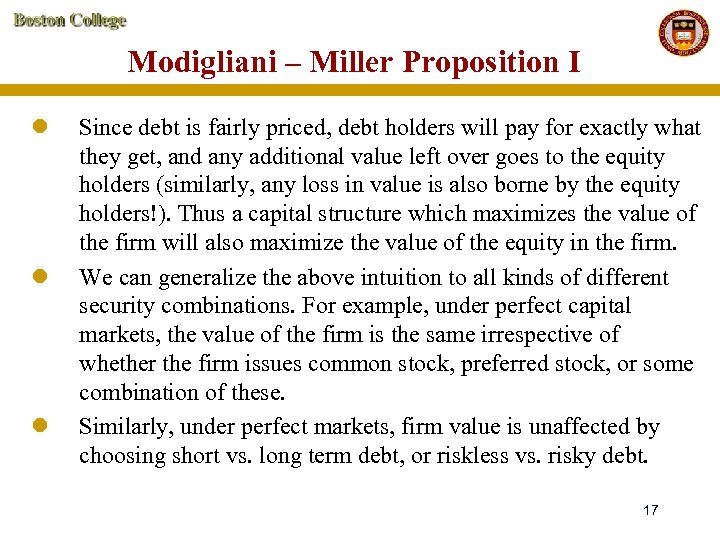Modigliani – Miller Proposition I l l l Since debt is fairly priced, debt holders will pay for exactly what they get, and any additional value left over goes to the equity holders (similarly, any loss in value is also borne by the equity holders!). Thus a capital structure which maximizes the value of the firm will also maximize the value of the equity in the firm. We can generalize the above intuition to all kinds of different security combinations. For example, under perfect capital markets, the value of the firm is the same irrespective of whether the firm issues common stock, preferred stock, or some combination of these. Similarly, under perfect markets, firm value is unaffected by choosing short vs. long term debt, or riskless vs. risky debt. 17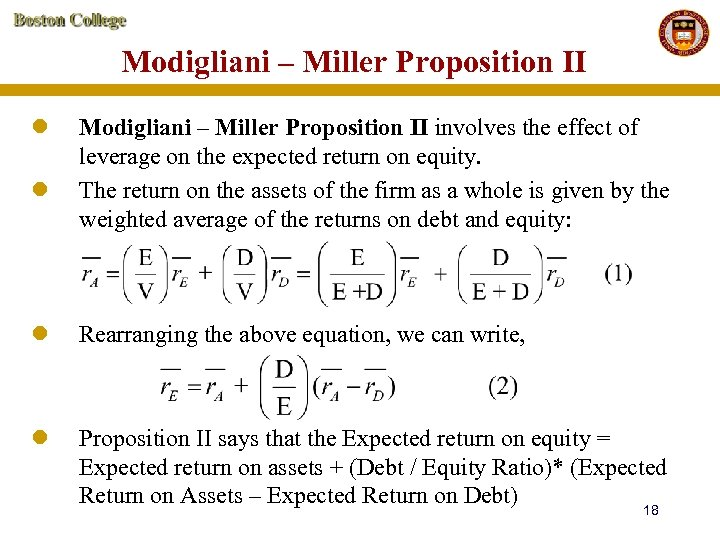Modigliani – Miller Proposition II l l Modigliani – Miller Proposition II involves the effect of leverage on the expected return on equity. The return on the assets of the firm as a whole is given by the weighted average of the returns on debt and equity: l Rearranging the above equation, we can write, l Proposition II says that the Expected return on equity = Expected return on assets + (Debt / Equity Ratio)* (Expected Return on Assets – Expected Return on Debt) 18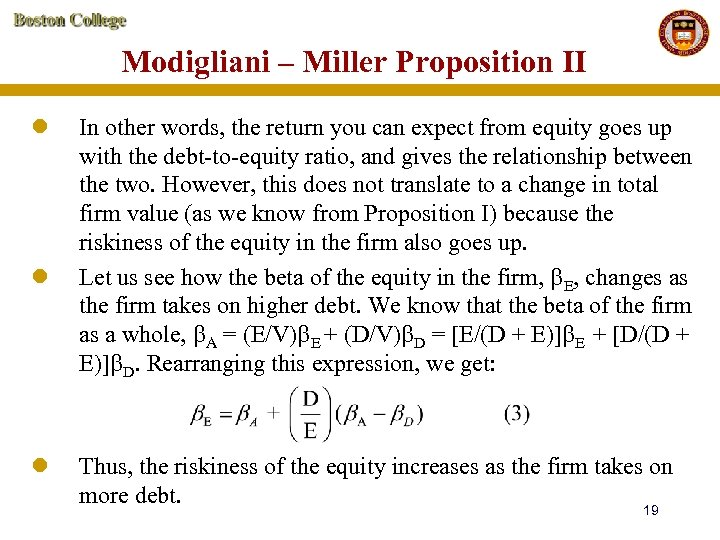Modigliani – Miller Proposition II l l l In other words, the return you can expect from equity goes up with the debt-to-equity ratio, and gives the relationship between the two. However, this does not translate to a change in total firm value (as we know from Proposition I) because the riskiness of the equity in the firm also goes up. Let us see how the beta of the equity in the firm, E, changes as the firm takes on higher debt. We know that the beta of the firm as a whole, A = (E/V) E + (D/V) D = [E/(D + E)] E + [D/(D + E)] D. Rearranging this expression, we get: Thus, the riskiness of the equity increases as the firm takes on more debt. 19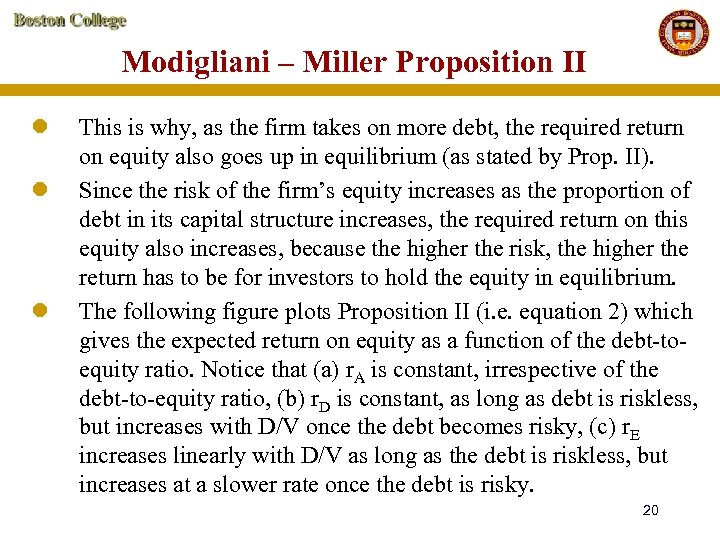Modigliani – Miller Proposition II l l l This is why, as the firm takes on more debt, the required return on equity also goes up in equilibrium (as stated by Prop. II). Since the risk of the firm’s equity increases as the proportion of debt in its capital structure increases, the required return on this equity also increases, because the higher the risk, the higher the return has to be for investors to hold the equity in equilibrium. The following figure plots Proposition II (i. e. equation 2) which gives the expected return on equity as a function of the debt-toequity ratio. Notice that (a) r. A is constant, irrespective of the debt-to-equity ratio, (b) r. D is constant, as long as debt is riskless, but increases with D/V once the debt becomes risky, (c) r. E increases linearly with D/V as long as the debt is riskless, but increases at a slower rate once the debt is risky. 20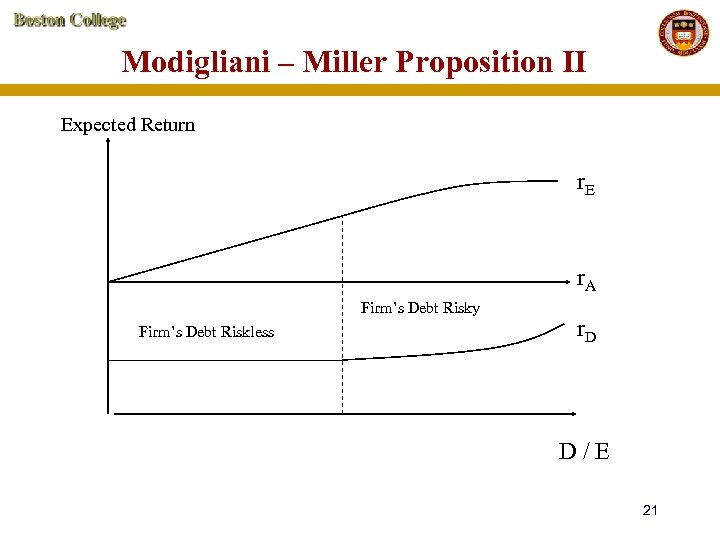Modigliani – Miller Proposition II Expected Return r. E r. A Firm’s Debt Risky Firm’s Debt Riskless r. D D/E 21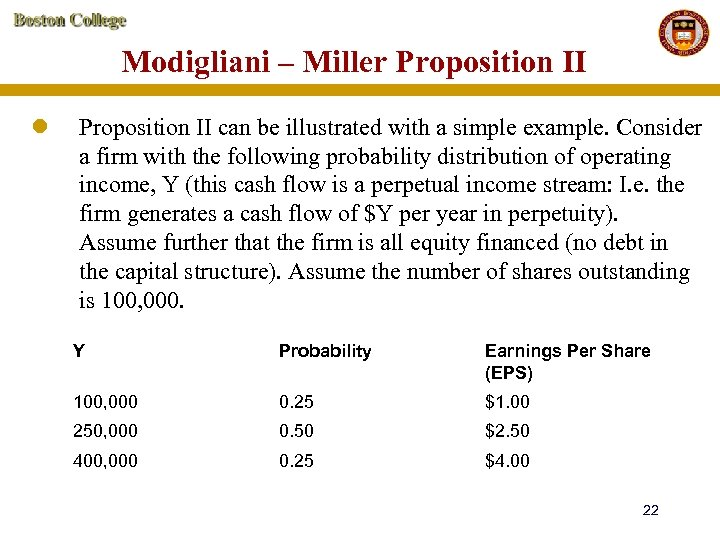Modigliani – Miller Proposition II l Proposition II can be illustrated with a simple example. Consider a firm with the following probability distribution of operating income, Y (this cash flow is a perpetual income stream: I. e. the firm generates a cash flow of \$Y per year in perpetuity). Assume further that the firm is all equity financed (no debt in the capital structure). Assume the number of shares outstanding is 100, 000. Y Probability Earnings Per Share (EPS) 100, 000 0. 25 \$1. 00 250, 000 0. 50 \$2. 50 400, 000 0. 25 \$4. 00 22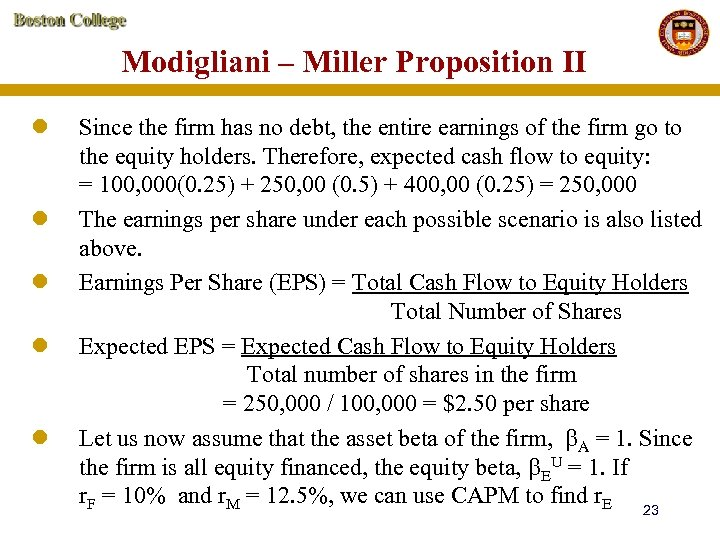Modigliani – Miller Proposition II l l l Since the firm has no debt, the entire earnings of the firm go to the equity holders. Therefore, expected cash flow to equity: = 100, 000(0. 25) + 250, 00 (0. 5) + 400, 00 (0. 25) = 250, 000 The earnings per share under each possible scenario is also listed above. Earnings Per Share (EPS) = Total Cash Flow to Equity Holders Total Number of Shares Expected EPS = Expected Cash Flow to Equity Holders Total number of shares in the firm = 250, 000 / 100, 000 = \$2. 50 per share Let us now assume that the asset beta of the firm, A = 1. Since the firm is all equity financed, the equity beta, EU = 1. If r. F = 10% and r. M = 12. 5%, we can use CAPM to find r. E 23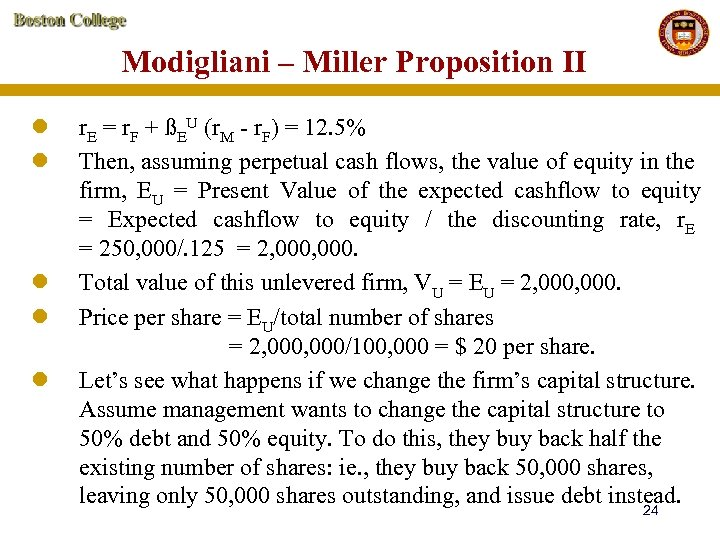Modigliani – Miller Proposition II l l l r. E = r. F + ßEU (r. M - r. F) = 12. 5% Then, assuming perpetual cash flows, the value of equity in the firm, EU = Present Value of the expected cashflow to equity = Expected cashflow to equity / the discounting rate, r. E = 250, 000/. 125 = 2, 000. Total value of this unlevered firm, VU = EU = 2, 000. Price per share = EU/total number of shares = 2, 000/100, 000 = \$ 20 per share. Let’s see what happens if we change the firm’s capital structure. Assume management wants to change the capital structure to 50% debt and 50% equity. To do this, they buy back half the existing number of shares: ie. , they buy back 50, 000 shares, leaving only 50, 000 shares outstanding, and issue debt instead. 24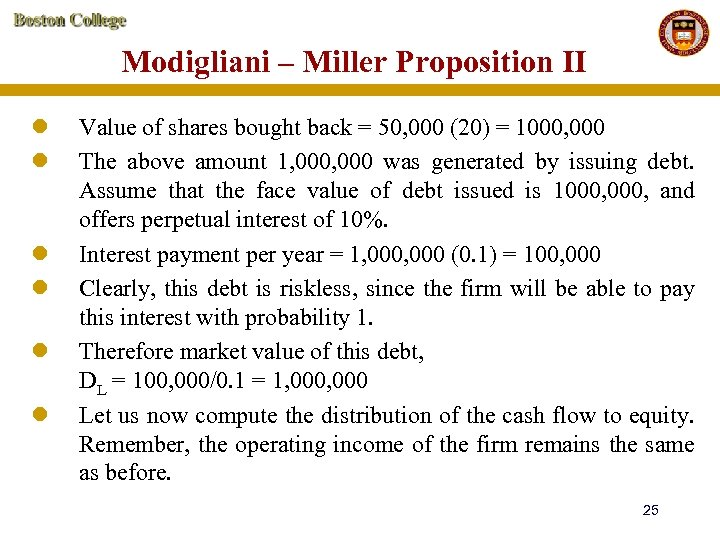Modigliani – Miller Proposition II l l l Value of shares bought back = 50, 000 (20) = 1000, 000 The above amount 1, 000 was generated by issuing debt. Assume that the face value of debt issued is 1000, and offers perpetual interest of 10%. Interest payment per year = 1, 000 (0. 1) = 100, 000 Clearly, this debt is riskless, since the firm will be able to pay this interest with probability 1. Therefore market value of this debt, DL = 100, 000/0. 1 = 1, 000 Let us now compute the distribution of the cash flow to equity. Remember, the operating income of the firm remains the same as before. 25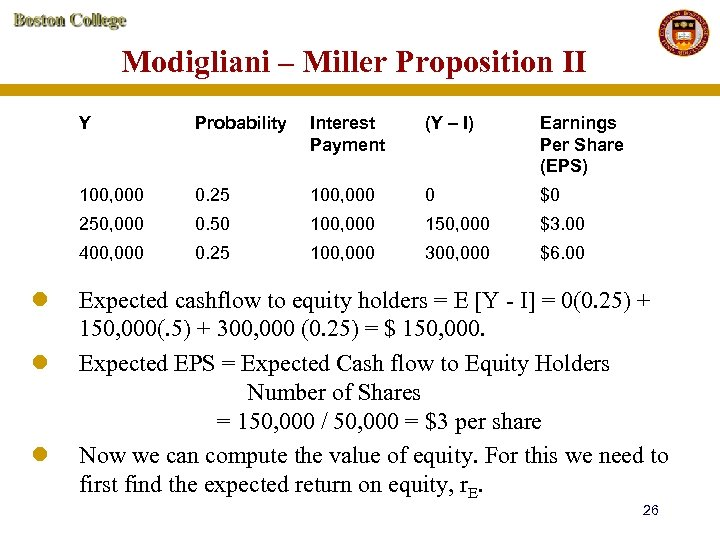Modigliani – Miller Proposition II Y Earnings Per Share (EPS) 0. 25 100, 000 0 \$0 0. 50 100, 000 150, 000 \$3. 00 400, 000 l (Y – I) 250, 000 l Interest Payment 100, 000 l Probability 0. 25 100, 000 300, 000 \$6. 00 Expected cashflow to equity holders = E [Y - I] = 0(0. 25) + 150, 000(. 5) + 300, 000 (0. 25) = \$ 150, 000. Expected EPS = Expected Cash flow to Equity Holders Number of Shares = 150, 000 / 50, 000 = \$3 per share Now we can compute the value of equity. For this we need to first find the expected return on equity, r. E. 26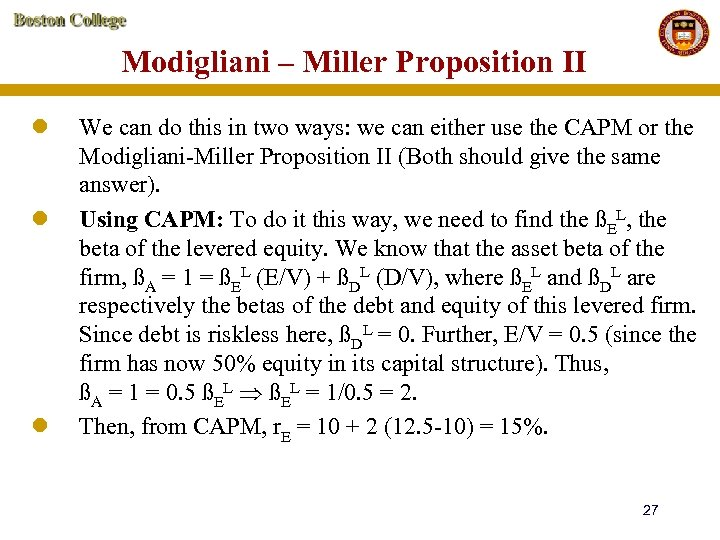Modigliani – Miller Proposition II l l l We can do this in two ways: we can either use the CAPM or the Modigliani-Miller Proposition II (Both should give the same answer). Using CAPM: To do it this way, we need to find the ßEL, the beta of the levered equity. We know that the asset beta of the firm, ßA = 1 = ßEL (E/V) + ßDL (D/V), where ßEL and ßDL are respectively the betas of the debt and equity of this levered firm. Since debt is riskless here, ßDL = 0. Further, E/V = 0. 5 (since the firm has now 50% equity in its capital structure). Thus, ßA = 1 = 0. 5 ßEL = 1/0. 5 = 2. Then, from CAPM, r. E = 10 + 2 (12. 5 -10) = 15%. 27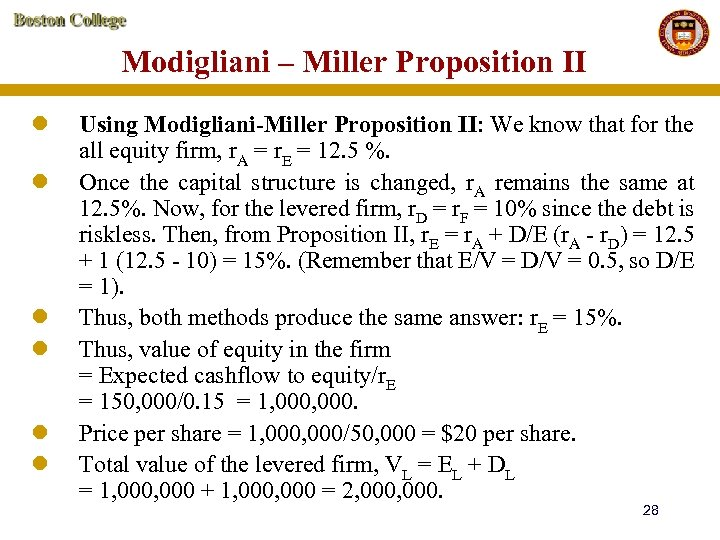Modigliani – Miller Proposition II l l l Using Modigliani-Miller Proposition II: We know that for the all equity firm, r. A = r. E = 12. 5 %. Once the capital structure is changed, r. A remains the same at 12. 5%. Now, for the levered firm, r. D = r. F = 10% since the debt is riskless. Then, from Proposition II, r. E = r. A + D/E (r. A - r. D) = 12. 5 + 1 (12. 5 - 10) = 15%. (Remember that E/V = D/V = 0. 5, so D/E = 1). Thus, both methods produce the same answer: r. E = 15%. Thus, value of equity in the firm = Expected cashflow to equity/r. E = 150, 000/0. 15 = 1, 000. Price per share = 1, 000/50, 000 = \$20 per share. Total value of the levered firm, VL = EL + DL = 1, 000 + 1, 000 = 2, 000. 28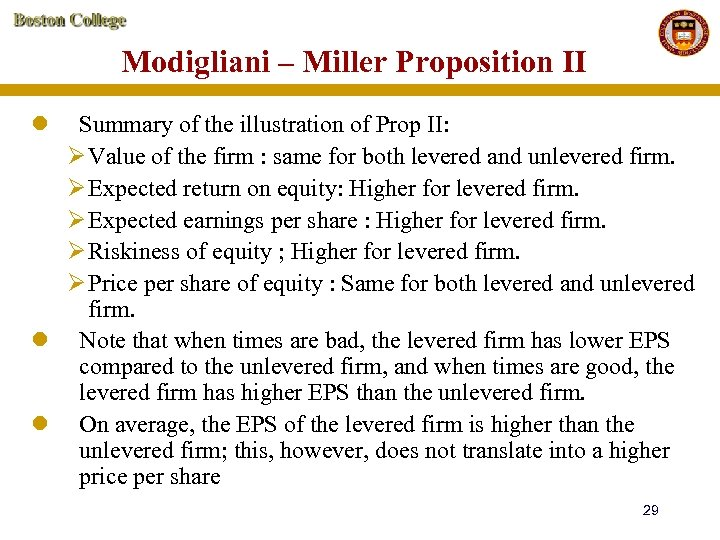Modigliani – Miller Proposition II l Summary of the illustration of Prop II: Ø Value of the firm : same for both levered and unlevered firm. Ø Expected return on equity: Higher for levered firm. Ø Expected earnings per share : Higher for levered firm. Ø Riskiness of equity ; Higher for levered firm. Ø Price per share of equity : Same for both levered and unlevered firm. l Note that when times are bad, the levered firm has lower EPS compared to the unlevered firm, and when times are good, the levered firm has higher EPS than the unlevered firm. l On average, the EPS of the levered firm is higher than the unlevered firm; this, however, does not translate into a higher price per share 29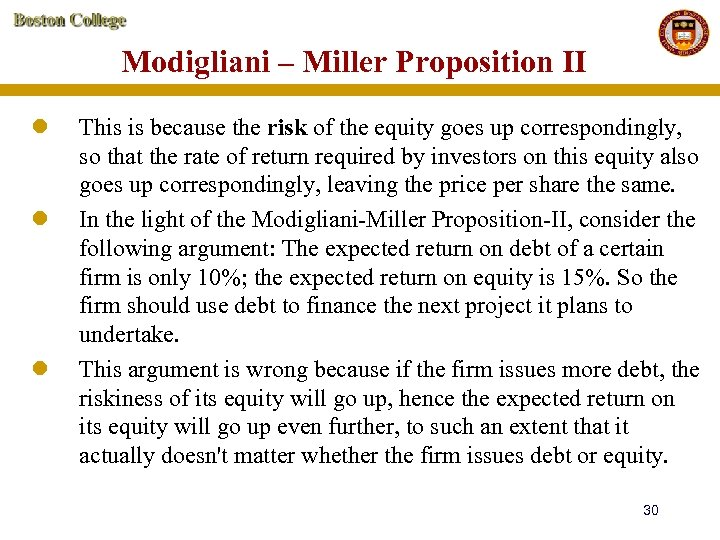Modigliani – Miller Proposition II l l l This is because the risk of the equity goes up correspondingly, so that the rate of return required by investors on this equity also goes up correspondingly, leaving the price per share the same. In the light of the Modigliani-Miller Proposition-II, consider the following argument: The expected return on debt of a certain firm is only 10%; the expected return on equity is 15%. So the firm should use debt to finance the next project it plans to undertake. This argument is wrong because if the firm issues more debt, the riskiness of its equity will go up, hence the expected return on its equity will go up even further, to such an extent that it actually doesn't matter whether the firm issues debt or equity. 30Modigliani – Miller Proposition II l l In fact, given the assumptions under which the Modigliani. Miller propositions hold (i. e. , ignoring taxes), the cost of capital remains the same irrespective of whether the firm issues debt or equity, and irrespective of the capital proportions of debt or equity used to finance its next project. Problem to be worked out at home: Company C is financed entirely by common stock and has an equity ß of 1. The stock of the company has a price earnings multiple of 10 and is priced to offer a 10% expected return. The company decides to repurchase half the common stock and substitute an equal value of debt. If the debt yields a risk-free 5%, and capital markets are perfect, then: 31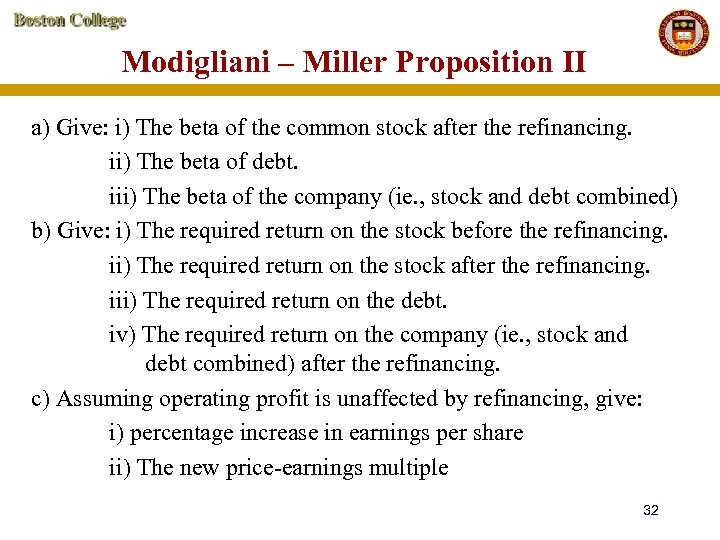Modigliani – Miller Proposition II a) Give: i) The beta of the common stock after the refinancing. ii) The beta of debt. iii) The beta of the company (ie. , stock and debt combined) b) Give: i) The required return on the stock before the refinancing. ii) The required return on the stock after the refinancing. iii) The required return on the debt. iv) The required return on the company (ie. , stock and debt combined) after the refinancing. c) Assuming operating profit is unaffected by refinancing, give: i) percentage increase in earnings per share ii) The new price-earnings multiple 32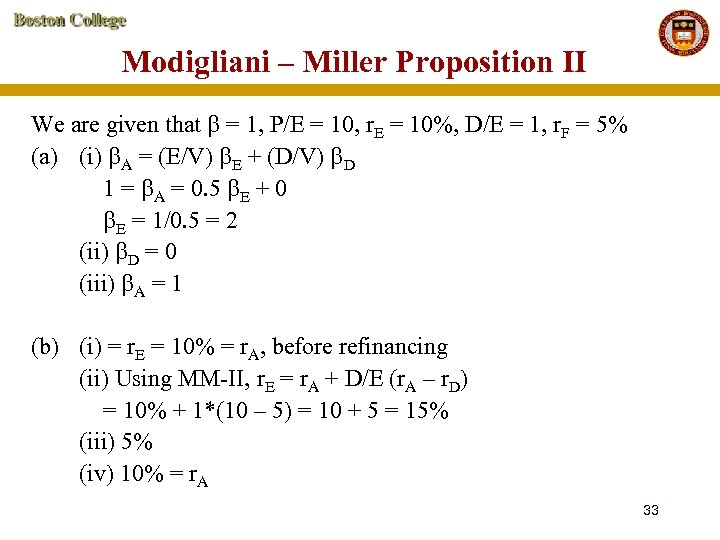Modigliani – Miller Proposition II We are given that = 1, P/E = 10, r. E = 10%, D/E = 1, r. F = 5% (a) (i) A = (E/V) E + (D/V) D 1 = A = 0. 5 E + 0 E = 1/0. 5 = 2 (ii) D = 0 (iii) A = 1 (b) (i) = r. E = 10% = r. A, before refinancing (ii) Using MM-II, r. E = r. A + D/E (r. A – r. D) = 10% + 1*(10 – 5) = 10 + 5 = 15% (iii) 5% (iv) 10% = r. A 33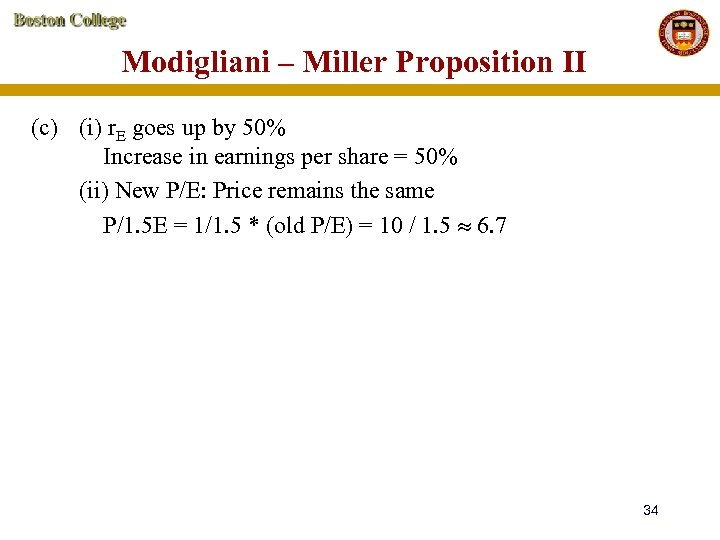Modigliani – Miller Proposition II (c) (i) r. E goes up by 50% Increase in earnings per share = 50% (ii) New P/E: Price remains the same P/1. 5 E = 1/1. 5 * (old P/E) = 10 / 1. 5 6. 7 34